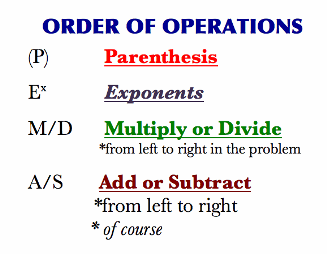# Order of operations

The order of operations is a very simple, but a very important rule. So important, in fact, that you will have to obide by it in every math problem you encounter – ever! It concernes the order in which these operations need to be performed. The order of operations lists mathematical operations in order in which they should be performed in a mathematical expression, from highest to lowest priority. Here is that list:The first letters of these operations (P-E-M-A) are often used as a mnemonic device to help you remember the order in which they should be performed. As you can see, operations inside parentheses have the highest priority, then exponents, then multiplication and, with the lowest priority, addition. To explain how this translates to problem solving, we will use this example:

(8 – 10)2 – (-9) * (-3)

This is a fairly complex example, but it contains all the elements needed to properly explain the order of operations . The first step we have to do is to get rid of the parentheses where it is possible. That can be done by solving the expressions inside the parentheses.

(-2)2 – (-9) * (-3)

Notice that we left -9 and -3 inside their parentheses. That is because there are no operations to perform there and we will get rid of them a bit later. We will leave them be to avoid clutter. Now we need to perform the exponentation, as it is second on the list of priorities. Since (-2)2 equals 4, we get:

4 – (-9) * (-3)

The next operation on the order of operations is multiplication, so let us do it. The result of multiplying (-9) and (-3) is 27, so we are left with:

4 – 27

The only operation left to perform now is subtraction, which has the lowest priority. The end result is:

-23

It is important to adhere to this order as it affects the end result. If we performed the operations in a different order, we could have gotten the wrong result.

If you wish to practice the order of operations, you can use the free worksheets below.

## Order of operations exams for teachers

 Exam Name File Size Downloads Upload date Non positive numeric expression Order of operations – non positive integers 725.4 kB 7057 September 3, 2019 Order of operations – non positive decimals 602.6 kB 3197 September 3, 2019 Order of operations – non positive fractions 901.4 kB 2964 September 3, 2019 Order of operations – non positive mixed numbers 933.8 kB 2646 September 3, 2019 Order of operations – non positive improper fractions 857.5 kB 2611 September 3, 2019 Positive numeric expression Order of operations – positive integers 662.2 kB 7443 September 3, 2019 Order of operations – positive decimal 732.4 kB 3095 September 3, 2019 Order of operations – positive fractions 848.8 kB 3623 September 3, 2019 Order of operations – positive mixed numbers 878.5 kB 2915 September 3, 2019 Order of operations – positive improper fractions 794.9 kB 2388 September 3, 2019 Non positive algebraic expression Order of operations – non positive integers 897.4 kB 3819 September 3, 2019 Order of operations – non positive decimals 897.4 kB 2262 September 3, 2019 Order of operations – non positive fractions 1.2 MB 1974 September 3, 2019 Order of operations – non positive mixed numbers 1 MB 2406 September 3, 2019 Order of operations – non positive improper fractions 1020.2 kB 2111 September 3, 2019 Positive algebraic expression Order of operations – positive integers 865.7 kB 4200 September 3, 2019 Order of operations – positive decimals 982.5 kB 2560 September 3, 2019 Order of operations – positive fractions 1.2 MB 2751 September 3, 2019 Order of operations – mixed fractions 1 MB 2492 September 3, 2019 Order of operations – improper fractions 981.2 kB 2423 September 3, 2019

## Order of operations worksheets for students

 Worksheet Name File Size Downloads Upload date Order of operations – Positive numeric expressions 781.9 kB 6891 September 3, 2019 Order of operations – Non positive numeric expressions 843.8 kB 4564 September 3, 2019 Order of operations – Positive algebraic expressions 924.8 kB 3943 September 3, 2019 Order of operations – Non positive algebraic expressions 1.4 MB 3644 September 3, 2019